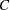Displayed Output

If you request the iteration history (ITPRINT), PROC PROBIT displays the following:

• the current value of the log likelihood

• the ridging parameter for the modified Newton-Raphson optimization process

• the current estimate of the parameters

• the current estimate of the parameterfor a natural (threshold) model

• the values of the gradient and the Hessian on the last iteration

If you include classification variables, PROC PROBIT displays the following:

• the numbers of levels for each classification variable

• the (ordered) values of the levels

• the number of observations used

After the model is fit, PROC PROBIT displays the following:

• the name of the input data set

• the name of the dependent variables

• the number of observations used

• the number of events and the number of trials

• the final value of the log-likelihood function

• the parameter estimates

• the standard error estimates of the parameter estimates

• approximate chi-square test statistics for the test

If you specify the COVB or CORRB options, PROC PROBIT displays the following:

• the estimated covariance matrix for the parameter estimates

• the estimated correlation matrix for the parameter estimates

If you specify the LACKFIT option, PROC PROBIT displays the following:

• a count of the number of levels of the response and the number of distinct sets of independent variables

• a goodness-of-fit test based on the Pearson’s chi-square

• a goodness-of-fit test based on the likelihood-ratio chi-square

If you specify only one independent variable, the normal distribution is used to model the probabilities, and the response is binary, then PROC PROBIT displays the following:

• the mean MU of the stimulus tolerance

• the scale parameter SIGMA of the stimulus tolerance

• the covariance matrix for MU, SIGMA, and the natural response parameterIf you specify the INVERSECL options, PROC PROBIT also displays the following:

• the estimated dose along with the 95% fiducial limits for probability levels 0.01 to 0.10, 0.15 to 0.85 by 0.05, and 0.90 to 0.99